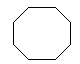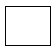Email us to get an instant 20% discount on highly effective K-12 Math & English kwizNET Programs!

#### Online Quiz (WorksheetABCD)

Questions Per Quiz = 2 4 6 8 10

### Grade 5 - Mathematics7.11 Regular Polygons

 A polygon in which all the sides are equal and all the angles are equal is called a regular polygon. Directions: Answer the following. Also draw at least five examples of regular polygon.
 Q 1: A regular polygon hassides of equal length and angles of equal measuresides of equal lengthangles of equal measure Q 2: The figure shown is a regularpentagonhexagonoctagon Q 3: A regular quadrilateral is also called asquareparallelogramrectangle Q 4: The figure shows regular triangle which is also calledisosceles triangleequilateral triangleright angle triangle Q 5: The figure shown is a regularpentagonsquareoctagon Q 6: The figure shown is a regularsquareoctagontriangle Question 7: This question is available to subscribers only! Question 8: This question is available to subscribers only!